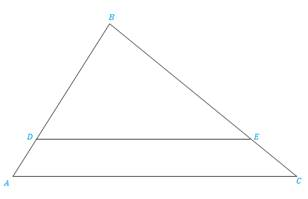Chapter 5.3, Problem 27EElementary Geometry For College St...

7th Edition
Alexander + 2 others
ISBN: 9781337614085

Solutions

Chapter
SectionElementary Geometry For College St...

7th Edition
Alexander + 2 others
ISBN: 9781337614085
Textbook Problem

In Exercises 25 to 28, Δ A B C ∼ Δ D B EExercises 25-28 Given: AC = 10, DE = 12, AD = 4. Find: DB

To determine

To find:

The side DB when the given sides are AC = 10, DE = 8, AD = 4 and ΔABCΔDBE.

Explanation

Definition:

CSSTP:

Corresponding sides of similar triangles are proportional.

Description:

The given figure is shown below.

Figure 1

From the given figure, it is observed that there are two triangles ABC and DBE and which is given that two triangles are similar.

Now draw the two triangles separately as shown below.

Figure 2

Figure 3

Since ΔABCΔDBE and from the definition of CSSTP, corresponding sides of similar triangles are proportional.

That is, BDBA=BEBC=DEAC.

It is given that AC = 10, DE = 8, AD = 4.

Find the side DB.

Consider the first and last two pairs of proportions DBAB=DEAC in order to find DB.

From Figure 1, it is observed that AB=AD+DB.

Still sussing out bartleby?

Check out a sample textbook solution.

See a sample solution

The Solution to Your Study Problems

Bartleby provides explanations to thousands of textbook problems written by our experts, many with advanced degrees!

Get Started

In Exercises 19-24, find the functions f + g, f g, fg, and fg. 23. f(x) = x+1x1; g(x) = x+2x2

Applied Calculus for the Managerial, Life, and Social Sciences: A Brief Approach

Sum of Square (SS), variance and standard deviation

Statistics for The Behavioral Sciences (MindTap Course List)

Simplify: 5251155

Elementary Technical Mathematics

Solve the equations in Exercises 126. 10x(x2+1)4(x3+1)510x2(x2+1)5(x3+1)4=0

Finite Mathematics and Applied Calculus (MindTap Course List)

True or False: The function whose graph is given at the right is a probability density function.

Study Guide for Stewart's Single Variable Calculus: Early Transcendentals, 8th

True or False: If and , then .

Study Guide for Stewart's Multivariable Calculus, 8th